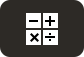# Free Pre-Algebra WorksheetsTutor-USA offers 100% Free Pre-Algebra Worksheets and printables for use by math teachers, students, and homeschool parents. Many of our Pre-Algebra worksheets contain an answer key and can be downloaded or printed, making them great for Pre-Algebra homework, classwork, or extra math practice.

Pre-Algebra is typically taught in grades 6, 7, 8 in middle school math courses. The overall goal of Pre-Algebra is to prepare the student for Algebra and beyond.

Pre-Algebra topics usually include natural numbers, integers, fractions, decimals, percents, exponents, factors, slope, properties of numbers, order of operations, and more. Students are also introduced to basic Algebra concepts such as variables and equations. Pre-Algebra students should be comfortable solving basic one or two step equations.

Our collection of free Pre-Algebra worksheets can be found below. They are free for use in any educational setting.

You can also browse Pre-Algebra Worksheets by Topic

In this free worksheet, students must determine if a a number is a palindrome, find multiples, test for divisibility, find factors, find GCF (Greatest common factor), and LCM (least common multiple).
Worksheet (Pre-Algebra)
Problems: 10
This free worksheet contains problems where students must write numbers in expanded form and index notation. Other problems include using leading number to estimate operations, write statements using...
Worksheet (Pre-Algebra)
Problems: 6
This free math worksheet contains problems on whole numbers and the number system. Students must write numbers in roman numerals, write numbers in arabic numerals, identify place values, write...
Worksheet (Pre-Algebra)
Problems: 6
In this free worksheet, students must use the coordinate plane to answer questions such as "In which quadrant does the point lie?", "Find the value of k if (k, 7) lies on the line y =...
Worksheet (Pre-Algebra)
Problems: 8
In this free algebra worksheet, students must factors polynomials expressions.
Worksheet (Pre-Algebra)
Problems: 15
In this free math worksheet, students must divide and simplify fractions and mixed numbers. All problems contain only numbers (no variables).
Worksheet (Pre-Algebra)
Problems: 15
This free algebra worksheet includes problems on solving equations involving two or more steps. Students must solve equations using inverse operations, the distributive property, and combining like...
Worksheet (Algebra)
Problems: 16
This free algebra worksheet begins with a overview of steps used to solve word problems. Students must then solve rectangle and consecutive integer word problems
Worksheet (Algebra)